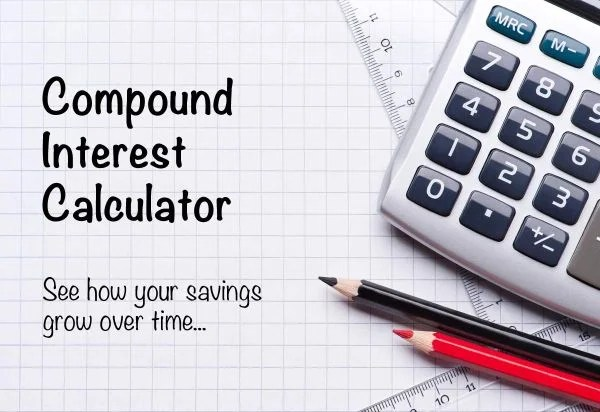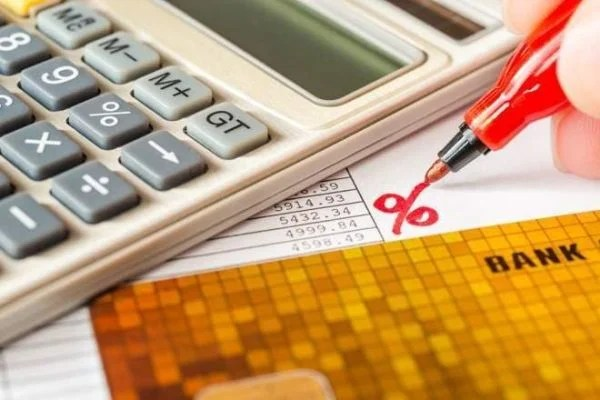How To Calculate Principal and Interest Payments?

How to calculate principal and interest payments?Here are some major steps that will help you in calculating the principal and interest payments such as:

• First of all, you have to write down your initial principal, your loan term and your APR. Convert your loan term and your APR to the point you want your payments. It is easy. For example, if your loan term is in the time period of years then you have to multiply it with the number 12 to get the monthly payment plan of your loan. And in the same way, you have to multiply your APR with 12 to get the interest payments.
• Add the values into an equation which should have a principal and interest payment and start performing the calculations after that.
• After the second step, multiply the periodic rate. It will help you in knowing the interest payment you have to pay for the first installment.
• Subtract the final number with monthly payment to know the number of principals.
• Now subtract the principal payment from the given loan principal and now start repeating the previous step with new principal balance.
• Constantly, repeat the process until the end of the loan payment term and till the principal balance is zero. You can make it at spreadsheet program or excel sheet. The spreadsheet will be showing the calculated amount of principal and interest payments in monthly loan payments.Basic information about interest payment and calculating principals

Interest payment is a payment you have to pay for the loan installments. It’s a payment amount that is determined by the interest rate on the account. For the investor, the interest payments are the incomes that are on cash accounts or the securities that are variable and fixed. A loan payment consists of two payments, one is principal payment and other is interest payment. So, the principal payment is a payment you have to pay towards the amount of payment you own.